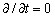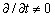# Kinematic models and piloted solving

## Introduction

The kinematic module of Flux includes three kinematic models: multi-static, imposed speed, or coupled load.

The piloted solving is different for each computation mode.

## Piloting the multi-static model

In the multi-static kinematic model, the moving part of the device is not moving. The computation of electromagnetic field is carried out for various arbitrary relative positions of moving and fixed parts.

This model performs a set of magneto-static computations (in Maxwell's equations), and does not take into consideration the dynamics equation. This model is equivalent to a parametrized study where the position of the moving part is a varying parameter.

The controlled solving is achieved through an intermediary parameter corresponding to the position of the moving part .

This model can be used with the Steady State AC magnetic application

## Piloting the imposed speed model

In the imposed speed kinematic model, the moving part is considered as moving at a constant velocity with respect to the fixed part.

The computation of electromagnetic field is carried out in stages at each time step, where the successive positions of the moving part are defined by the imposed speed of the moving part. As in the previous kinematic model, the mechanical equations are not considered.

In the imposed speed kinematic model, the physical application used is the transient magnetic physical application. In this case, the Maxwell's equations consider the time dependence of the electromagnetic field ().

The controlled solving is carried out with one of two intermediary parameters:

• the time parameter or
• the position parameter

## Piloting the coupled load model

In the coupled load kinematic model, the moving part drives an external device that represents the mechanical load of the studied device.

This is the model in which the magneto-mechanical coupling is considered, that is to say, both the magnetic aspects and the kinematic aspects of a problem. The physical application used is the transient magnetic physical application.

The piloted solving is carried out with the time parameter.